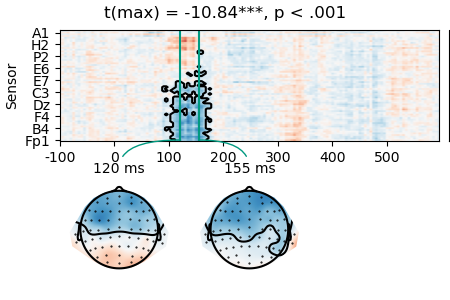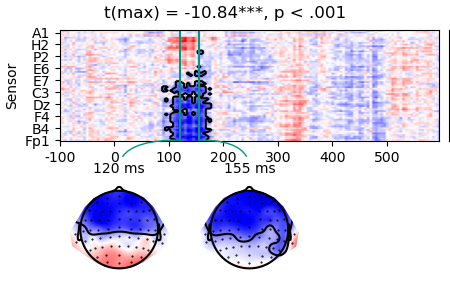# Two-stage test

When trials are associated with continuous predictor variables, averaging is often a poor solution that loses part of the data. In such cases, a two-stage design can be employed that allows using the continuous predictor variable to test hypotheses at the group level. A two-stage analysis involves:

• Stage 1: fit a regression model to each individual subject’s data

• Stage 2: test regression coefficients at the group level

The example uses the same simulated data and design used in Multiple regression. The data are meant to vaguely resemble data from a word reading experiment, but not intended as a physiologically realistic simulation.

```# sphinx_gallery_thumbnail_number = 1
from eelbrain import *
```

## Stage 1

Generate simulated data: each function call to `datasets.simulate_erp()` generates a dataset for one subject (in a real experiment this would be replaced with a function that loads data for this subject). For each subject, a multiple regression model is fit using n characters and cloze probability as continuous predictor variables.

```lms = []
for subject in range(10):
# generate data for one subject
ds = datasets.simulate_erp(seed=subject)
# Re-reference EEG data
ds['eeg'] -= ds['eeg'].mean(sensor=['M1', 'M2'])
# Fit stage 1 model (samples=0 because we do not need permutations at stage 1)
lm = testnd.LM('eeg', 'n_chars + cloze', data=ds, samples=0, subject=str(subject))
lms.append(lm)
```

## Stage 2

Prepare a `Dataset` with the first level statistic of interest.

```rows = []
for lm in lms:
rows.append([lm.subject, lm.t('intercept'), lm.t('n_chars'), lm.t('cloze')])
# When creating the dataset for stage 2 analysis, declare subject as random factor;
# this is only relevant if performing ANOVA as stage 2 test.
data = Dataset.from_caselist(['subject', 'intercept', 'n_chars', 'cloze'], rows, random='subject')
data
```

Now we can test whether the first stage estimates are consistent across subject.

```result = testnd.TTestOneSample('n_chars', data=data, pmin=0.05, tstart=0, tstop=0.300)
p_cb = p.plot_colorbar(right_of=p.axes, label='t')
``````Permutation test:   0%|          | 0/1023 [00:00<?, ? permutations/s]
Permutation test:  16%|█▌        | 164/1023 [00:00<00:00, 1628.08 permutations/s]
Permutation test:  34%|███▍      | 348/1023 [00:00<00:00, 1750.81 permutations/s]
Permutation test:  52%|█████▏    | 532/1023 [00:00<00:00, 1789.51 permutations/s]
Permutation test:  71%|███████   | 722/1023 [00:00<00:00, 1829.56 permutations/s]
Permutation test:  89%|████████▉ | 914/1023 [00:00<00:00, 1859.94 permutations/s]
Permutation test: 100%|██████████| 1023/1023 [00:00<00:00, 1829.95 permutations/s]
```

Instead of t-values, we might want to visualize regression coefficients:

```rows = []
for lm in lms:
rows.append([lm.subject, lm.coefficient('n_chars')])
data_c = Dataset.from_caselist(['subject', 'n_chars'], rows, random='subject')